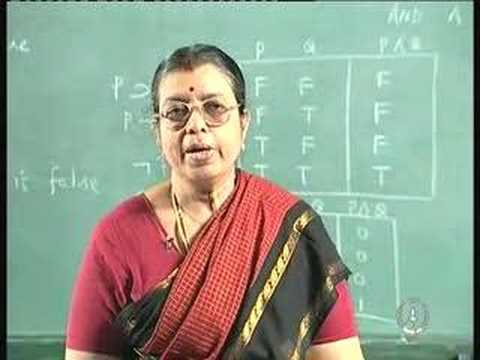NPTEL Courses

# Discrete Mathematical Structures

This course deals with topics about discrete mathematical structures. Discrete Mathematics is a study of discrete structures which are abstract mathematical models dealing with discrete objects and their relationship between them. This course covers lessons in logic, sets, relations, functions, graphs, combinatorics, recurrence relations, algebras and finite state automaton. The aim of this course is not only make people learn about these topics, but also help them to develop the habit of thinking mathematically. (from nptel.ac.in )

Instructor

Computer science
2007

#### Lecture 01 - Propositional Logic